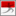Rob Whitney quantum physics of nanostructures — theoretical physics Laboratoire de Physique et Modélisation des Milieux Condensés Université Grenoble Alpes & CNRS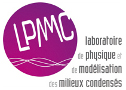HOME INTRO EVENTS PAPERS TALKS PAST LINKS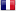FRANCAIS
 Written in 2010 (with addition in 2012) A BRIEF INTRODUCTION TO QUANTUM MECHANICS, QUANTUM CHAOS & DECOHERENCE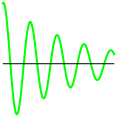Quantum mechanics is the theory of wave-particle duality; it tells us that a particle - such as an electron - moves as a wave. It spreads out and goes to many places at once. If part of that wave crosses another part, (perhaps after reflecting off something), then the two parts of the wave interferes (like light interferes in the well-known Young's double-slit experiment). The electron is never found in the places where destructive interference occurs. Waves can also go around and through small obstacles because they rarely have a well-defined position or energy. The position-momentum uncertainty principle means waves can diffract around obstacles, while the energy-time uncertainty principle means they can tunnel through obstacles. Finally quantum waves have an additional property that other waves do not, they can become entangled with each other.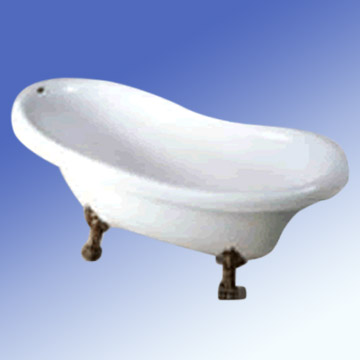A real bath tub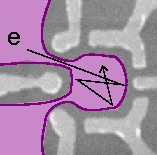A quantum dot : a nano-scale "bath tub" of electrons Marcus Lab (2003) QUANTUM CHAOS Quantum mechanic plus chaos theory. Chaos theory tells us that a billiard-ball rolling around in a bath follows a chaotic path. This means that any error you make in measuring the initial postion or velocity of the ball will lead to an error in your prediction of its motion which grows exponentially with time, until fairly soon your prediction becomes completely wrong! In such cases, one can use chaos theory to make statistical predictions (i.e. "there is a 10% chance of the ball going there") much like a weather forecast (i.e. "there is a 10% chance of rain"). Quantum mechanics tells us that electrons (unlike billiard-balls) move as waves. Imagine filling the bath with water and looking at how ripples move on the surface of the water. The bath is the same, but now you are studying how waves (not particles) move within it. These waves do many things that particles (such as billiard balls) do not. They go to many places at once. They diffract, tunnel and interfere. Electrons in a typical semiconductor quantum dot do the same. We ask how the wave nature of electrons affects their chaotic motion. Using the semiclassical limit of Feynman's path-integrals (the "ray-optics" limit), we go beyond "Berry's diagonal approximation" to look at interference and other wave effects. I have been particularly interested in particle flow through open quantum chaotic systems. I study how quantum chaos induces interference effects such as weak-localization and conductance fluctuations, and have proposed huge interference effects in quantum chaotic systems with a discrete symmetry (mirror-symmetry). I have also study quantum noise in such systems. Added in 2012. Since 2011, I have mainly worked to understand thermoelectric effects associated with such quantum particle flows (when an electrical current flow induces a heat flow, and vice versa). I am particularly interested in the connection between this and quantum thermodynamics.Quantum oscillations decay irreversibility due to decoherence IRREVERSIBILITY: QUANTUM DECOHERENCE Returning to the ripples in the bath, you will notice that they quickly dissappear. It is hard to make a ripple travel from one end of the bath to the other end and back. The friction between water molecules destroys the wave (converting its energy into heat). In contrast, electrons cannot dissappear so their waves do not decay. However each electron does interact with other particles nearby. The "friction" between the electron ond other particles leads to something called decoherence or dephasing of the wave. The wave becomes entangled with the waves of other particles and its phase gets lost. Then the wave still exists, but when it encounters another part of the wave it does not make an interference pattern. This decoherence process has particularly stark effects when the system has a finite number of discrete levels. This occurs when the wavelength is similar to the container's size, then the wave must be made of a small number of discrete standing waves. Another example of discrete levels is the electron's spin in a magnetic field; it is a two-level system. Here I ask how the type of interaction with other particles (or exsternal noise) affects the nature of the decoherence. In particular I study: The decoherence of geometric phases Spin decoherence in quantum wires: "Dephasing diode" Decoherence by coloured-noise (non-zero memory times) Anderson orthogonality catastrophies induced by environments of noise
 Design/maintenance: Rob Whitney   Hosting: www.free.fr